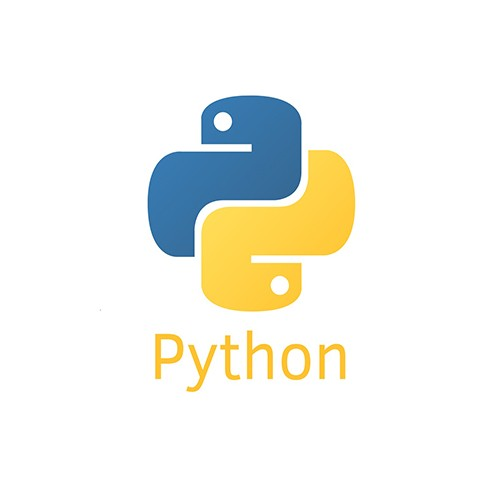# python如何获取程序执行时间?• yang   2020-05-22 13:37:53820python获取程序执行时间的方法：

1、使用time.clock()方法获取程序执行时间

```import time
start =time.clock()
#中间写上代码块
end = time.clock()
print('Running time: %s Seconds'%(end-start))```

2、使用time.time()方法计算

```import time
start=time.time()
#中间写上代码块
end=time.time()
print('Running time: %s Seconds'%(end-start))```

3、使用datetime.datetime.now()方法获取

```import datetime
start=datetime.datetime.now()
#中间写代码块
end=datetime.datetime.now()
print('Running time: %s Seconds'%(end-start))```